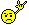# RGB bypass mod for Neo models 5 to 7

#### RockstarRunner

##### Wind Jammin' All Night",
Hey,
Here is some info for anyone wanting to attempt the RGB bypass fix on a model 5 to 7 series NeoGeo.

I would like to point out all technical information was provided by forum member MKLBigger image

This picture shows the under side of the Neo mainboard, the corner that is under the A/V connector.
There are 4 connections to make as detailed in the image, one for each R,G,B and another for the true sync.
These connections should be made with an 82ohm resistor between each of the RGB lines(sync line does not require the resistor), as the standard signal strength is too intense for the t.v. to display correctly.
Connections are taken from any of the 7 resistors for a given colour, the points shown in mine are not required, just what I went with.
Note that the bottom plastic shell has an indented vent near the point where you're doing the mod so make sure you're soldering the wires in such a way that you can fit the board back into the shell.
Connections are made to the ferrite beads (the blue things) just before the A/V port pins instead of the A/V port pins themselves, so the signals will be protected from possible RFI/EMI interferences.Bigger image

Remove the labelled components, that being 3 resistors & 4 capacitors.

After having done this mod, the vertical lines that where present accross the screen are now gone, the colours are vibrant, and the image is sharp and clear.
I highly recommend doing this mod, it made a world of difference to my Neo, serial #333883

Note: resistor order when viwed from the top of the board
01 = 220 (R)
02 = 470 (R)
03 = 3.9k (R)
04 = 2.2k (R)
05 = 1k (R)
06 = 150 (R)
07 = 8.2k (R)
08 = 220 (G)
09 = 470 (G)
10 = 150 (G)
11 = 8.2k (G)
12 = 1k (G)
13 = 3.9k (G)
14 = 2.2k (G)
15 = 3.9k (B)
16 = 2.2k (B)
17 = 1k (B)
18 = 470 (B)
19 = 220 (B)
20 = 150 (B)
21 = 8.2k (B)

Note: After having done this mod, your NeoGeo will no longer display composite out video! only RGB.

If you have any technical questions, not answered here, I recommend contacting MKL, he's the man in this area!
And one last point, only do this if you have a steady hand, those solder points are quite close together.

Last edited: